6306.0 - Employee Earnings and Hours, Australia, May 2006
ARCHIVED ISSUE Released at 11:30 AM (CANBERRA TIME) 28/02/2007
 Page tools: .mffeedback,#pjs { display :none; } Enable Javascript to Print PagesPrint All TECHNICAL NOTE SAMPLING ERROR RELIABILITY OF ESTIMATES 1 As the estimates in this publication are based on information relating to a sample of employers and employees, rather than a full enumeration, they are subject to sampling variability, that is, they may differ from the figures that would have been produced if the data had been obtained from all employers and all employees. The difference, called sampling error, should not be confused with inaccuracy that may occur because of imperfections in reporting by respondents or in processing by the ABS. Such inaccuracy is referred to as non-sampling error and may occur in any enumeration whether it be a full count or a sample. Efforts have been made to reduce non-sampling error by careful design of questionnaires, detailed checking of returns and quality control of processing. 2 The sampling error associated with any estimate can be estimated from the sample results. One measure of sampling error is given by the standard error, which indicates the degree to which an estimate may vary from the value that would have been obtained from a full enumeration (the ‘true value'). There are about two chances in three that a sample estimate differs from the true value by less than one standard error, and about nineteen chances in twenty that the difference will be less than two standard errors. 3 An example of the use of a standard error is as follows. From table 1, the estimated average weekly total cash earnings for all male employees in Australia is \$1,020.30, with a standard error of \$9.50 (from table 22). Then there would be about two chances in three that a full enumeration would have given an estimate in the range \$1,010.80 to \$1,029.80 and about nineteen chances in twenty that it would be in the range \$1,001.30 to \$1,039.30. 4 The difference between two survey estimates is also an estimate and it is therefore subject to sampling variability. The standard error on the difference between two survey estimates in the one time period (i.e. x-y) can be calculated using the following formula: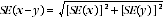5 The formula above will overestimate the standard error where there is a positive correlation between two estimates (e.g. male and female school teachers). While this formula will only be accurate where there is no correlation between two estimates (e.g. estimates from different states), it is expected to provide a reasonable approximation of the standard error for the difference between two survey estimates. 6 From table 1, the estimated average weekly total cash earnings for all female employees in Australia is \$677.80, with a standard error of \$7.60 (from table 22). The difference between the earnings of male and female employees is \$342.50. The estimate of the standard error of the difference between the average weekly total cash earnings for male and female employees in Australia is: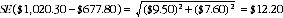7 There are about two chances in three that the true figure for the difference between male and female average weekly total cash earnings lies in the range \$330.30 to \$354.70, and about nineteen chances in twenty that the figure is in the range \$318.10 to \$366.90. 8 The formula above can be used to estimate the standard error on a difference between estimated averages in two different years. (The movement standard error will be approximately 1.4 times the standard error on the level estimate, if the standard errors on the two level estimates are similar.) 9 Another measure of the sampling error is the relative standard error, which is obtained by expressing the standard error as a percentage of the estimate. Both the standard error and relative standard error are used to measure the reliability of estimates. 10 Relative standard errors can be calculated using the actual standard error and the survey estimate using the formula below: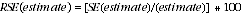11 For example, from table 1, the average weekly total cash earnings for all male employees in Australia is \$1,020.30, and for all female employees it is \$677.80. Table 22 shows an estimate for the standard error on the male estimate is \$9.50, and an estimate of the standard error on the female estimate is \$7.60. 12 Applying the above formula the relative standard errors for the average weekly total cash earnings for all male employees and all female employees can be worked out as follows: All male employees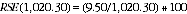All female employees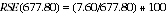13 An asterisk appears against an estimate in this publication where the sampling variability is considered high. For the tables in this publication, estimates with relative standard errors between 25% and 50% have been labelled with a single asterisk; estimates with a relative standard error greater than 50% have been labelled with a double asterisk. 14 Standard errors can be used to construct confidence intervals around the estimated proportions. There are about two chances in three that the 'true' value is within the interval that ranges from the sample estimate minus one standard error (estimate - 1xSE) to the sample estimate plus one standard error (estimate + 1xSE). There are approximately 19 chances in 20 that the 'true' value lies within the interval from the estimate minus two standard errors (estimate - 2xSE) to the estimate plus two standard errors (estimate + 2xSE). 15 The above rule gives a symmetric confidence interval that is reasonably accurate when the estimated proportion is not too near 0.00 or 1.00. Where the estimated proportion is close to 0.00 or 1.00 it would be more accurate to use a confidence interval that was not symmetric around the sample estimate. If an estimate is close to 1.00, then the upper boundary of the confidence interval should be closer to the sample estimate than suggested above, while the lower boundary should be further from the sample estimate. Similarly, if an estimate is close to 0.00, then the lower boundary of the confidence interval should be closer to the sample estimate than suggested above, while the upper boundary should be further from the sample estimate. In particular, the symmetric confidence interval could include values that are not between 0.00 and 1.00. In such a case a good rule of thumb is to use a confidence interval of the same size as the symmetric one, but with the lower (or upper) boundary set to 0.00 (or 1.00). 16 Tables 22 to 26 contain estimates of standard errors from which confidence intervals may be constructed. Document Selection These documents will be presented in a new window.